## Math & Trigonometry - Lumos Educational App Store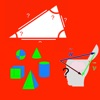3.61
> Price -Free
\$0

#### DESCRIPTION:

Used in more than 250 countries! With this application, math becomes easy thanks to all these functions and details of calculations. Available calculations:      -Rectangle triangle      -Pythagore      Triangle      -Trigonometry      -Calculating volume      -Calculated inverted volume      -Simplification of fractions      - Area calculation      -Inverted area calculation      - Calculation of Thales      - Perimeter calculation      -Inverse perimeter calculation      -Scaling steps      - Derivatives      -And more others ...

#### OVERVIEW:

Math & Trigonometry is a free educational mobile app By Maxime Haeyaert.It helps students practice the following standards .

This page not only allows students and teachers download Math & Trigonometry but also find engaging Sample Questions, Videos, Pins, Worksheets, Books related to the following topics.

Developer: Maxime Haeyaert

Developer URL: NA

Software Version: 8.0

Category: Education

Release Date: 2017-08-24T15:51:40Z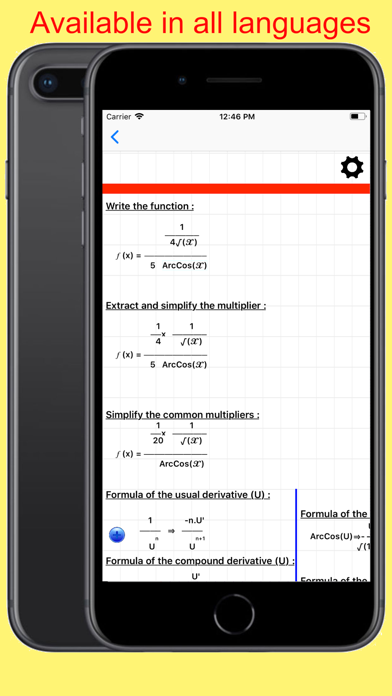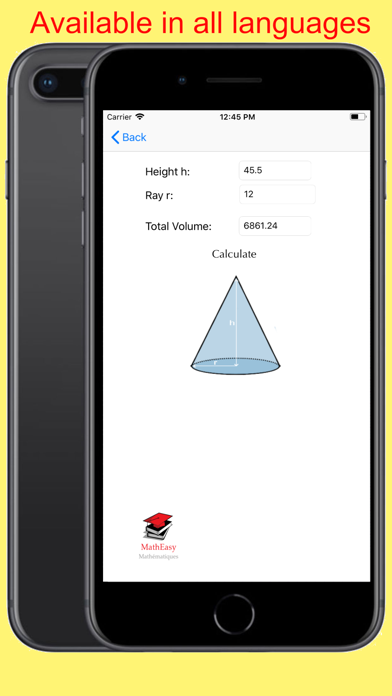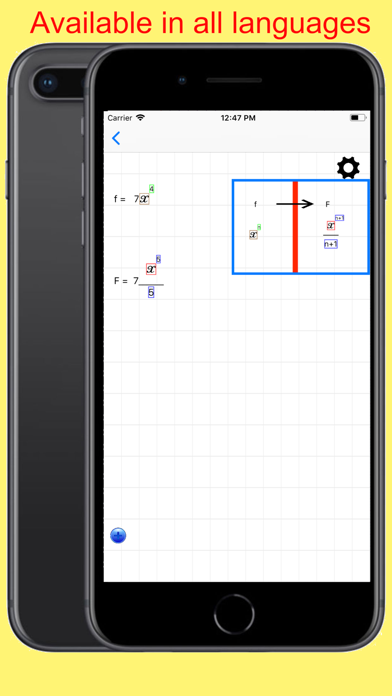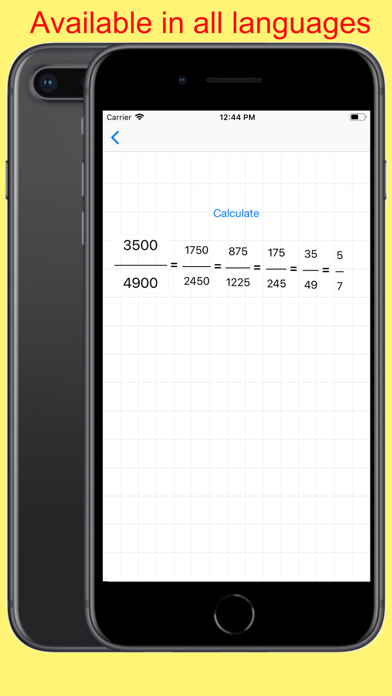#### Are you the Developer?EdSearch WebSearch